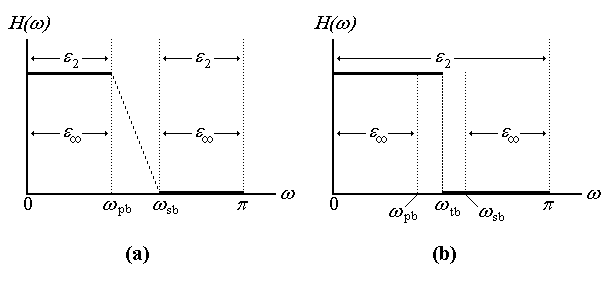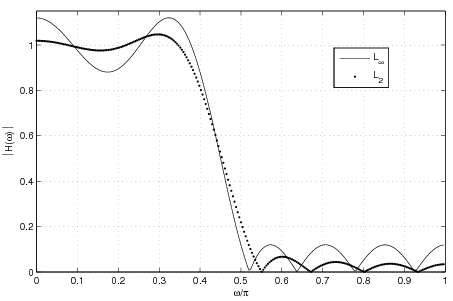# 0.10 Constrained least squares (cls) problem  (Page 2/5)

 Page 2 / 5

## Two problem formulations

As mentioned in [link] , one can address problem [link] in two ways depending on how one views the role of the transition band in a CLS problem. The original problem posed by Adams in [link] can be written as follows,

$\begin{array}{cc}\underset{h}{\text{min}}\hfill & {\parallel D\left(\omega \right)-H\left(\omega ;h\right)\parallel }_{2}\hfill \\ \text{subject}\phantom{\rule{4.pt}{0ex}}\text{to}\hfill & |D\left(\omega \right)-H\left(\omega ;h\right)|\phantom{\rule{-0.166667em}{0ex}}\le \phantom{\rule{-0.166667em}{0ex}}\tau \phantom{\rule{1.em}{0ex}}\forall \phantom{\rule{0.277778em}{0ex}}\omega \in \left[0,{\omega }_{pb}\right]\cup \left[{\omega }_{sb},\pi \right]\hfill \end{array}$

where $0\phantom{\rule{-0.166667em}{0ex}}<\phantom{\rule{-0.166667em}{0ex}}{\omega }_{pb}\phantom{\rule{-0.166667em}{0ex}}<\phantom{\rule{-0.166667em}{0ex}}{\omega }_{sb}\phantom{\rule{-0.166667em}{0ex}}<\phantom{\rule{-0.166667em}{0ex}}\pi$ . From a traditional standpoint this formulation feels familiar. It assigns fixed frequencies to the transition band edges as a number of filter design techniques do. As it turns out, however, one might not want to do this in CLS design.

An alternate formulation to [link] could implicitly introduce a transition frequency ${\omega }_{tb}$ (where ${\omega }_{pb}\phantom{\rule{-0.166667em}{0ex}}<\phantom{\rule{-0.166667em}{0ex}}{\omega }_{tb}\phantom{\rule{-0.166667em}{0ex}}<\phantom{\rule{-0.166667em}{0ex}}{\omega }_{sb}$ ); the user only specifies ${\omega }_{tb}$ . Consider

$\begin{array}{ccc}\underset{h}{\text{min}}\hfill & {\parallel D\left(\omega \right)-H\left(\omega ;h\right)\parallel }_{2}\hfill & \forall \phantom{\rule{0.277778em}{0ex}}\omega \in \left[0,\pi \right]\hfill \\ \text{subject}\phantom{\rule{4.pt}{0ex}}\text{to}\hfill & |D\left(\omega \right)-H\left(\omega ;h\right)|\phantom{\rule{-0.166667em}{0ex}}\le \phantom{\rule{-0.166667em}{0ex}}\tau \hfill & \forall \phantom{\rule{0.277778em}{0ex}}\omega \in \left[0,{\omega }_{pb}\right]\cup \left[{\omega }_{sb},\pi \right]\hfill \end{array}$

The algorithm at each iteration generates an induced transition band in order to satisfy the constraints in [link] . Therefore $\left\{{\omega }_{pb},{\omega }_{sb}\right\}$ vary at each iteration.Two formulations for Constrained Least Squares problems.

It is critical to point out the differences between [link] and [link] . [link] .a explains Adams' CLS formulation, where the desired filter response is only specified at the fixed pass and stop bands. At any iteration, Adams' method attempts to minimize the least squares error ( ${\epsilon }_{2}$ ) at both bands while trying to satisfy the constraint $\tau$ . Note that one could think of the constraint requirements in terms of the Chebishev error ${\epsilon }_{\infty }$ by writing [link] as follows,

$\begin{array}{cc}\underset{h}{\text{min}}\hfill & {\parallel D\left(\omega \right)-H\left(\omega ;h\right)\parallel }_{2}\hfill \\ \text{subject}\phantom{\rule{4.pt}{0ex}}\text{to}\hfill & {\parallel D\left(\omega \right)-H\left(\omega ;h\right)\parallel }_{\infty }\phantom{\rule{-0.166667em}{0ex}}\le \phantom{\rule{-0.166667em}{0ex}}\tau \phantom{\rule{1.em}{0ex}}\forall \phantom{\rule{0.277778em}{0ex}}\omega \in \left[0,{\omega }_{pb}\right]\cup \left[{\omega }_{sb},\pi \right]\hfill \end{array}$

In contrast, [link] .b illustrates our proposed problem [link] . The idea is to minimize the least squared error ${\epsilon }_{2}$ across all frequencies while ensuring that constraints are met in an intelligent manner. At this point one can think of the interval $\left({\omega }_{pb},{\omega }_{sb}\right)$ as an induced transition band, useful for the purposes of constraining the filter. [link] presents the actual algorithms that solve [link] , including the process of finding $\left\{{\omega }_{pb},{\omega }_{sb}\right\}$ .

It is important to note an interesting behavior of transition bands and extrema points in ${l}_{2}$ and ${l}_{\infty }$ filters. [link] shows ${l}_{2}$ and ${l}_{\infty }$ length-15 linear phase filters (designed using Matlab's firls and firpm functions); the transition band was specified at $\left\{{\omega }_{pb}=0.4/\pi ,{\omega }_{sb}=0.5/\pi \right\}$ . The dotted ${l}_{2}$ filter illustrates an important behavior of least squares filters: typically the maximum error of an ${l}_{2}$ filter is located at the transition band. The solid ${l}_{\infty }$ filter shows why minimax filters are important: despite their larger error across most of the bands, the filter shows the same maximum error at all extrema points, including the transition band edge frequencies. In a CLS problem then, typically an algorithm will attempt to reduce iteratively the maximum error (usually located around the transition band) of a series of least squares filters.Comparison of l 2 and l ∞ filters.

Another important fact results from the relationship between the transition band width and the resulting error amplitude in ${l}_{\infty }$ filters. [link] shows two ${l}_{\infty }$ designs; the transition bands were set at $\left\{0.4/\pi ,0.5/\pi \right\}$ for the solid line design, and at $\left\{0.4/\pi ,0.6/\pi \right\}$ for the dotted line one. One can see that by widening the transition band a decrease in error ripple amplitude is induced.

#### Questions & Answers

Is there any normative that regulates the use of silver nanoparticles?
Damian Reply
what king of growth are you checking .?
Renato
What fields keep nano created devices from performing or assimulating ? Magnetic fields ? Are do they assimilate ?
Stoney Reply
why we need to study biomolecules, molecular biology in nanotechnology?
Adin Reply
?
Kyle
yes I'm doing my masters in nanotechnology, we are being studying all these domains as well..
Adin
why?
Adin
what school?
Kyle
biomolecules are e building blocks of every organics and inorganic materials.
Joe
anyone know any internet site where one can find nanotechnology papers?
Damian Reply
research.net
kanaga
sciencedirect big data base
Ernesto
Introduction about quantum dots in nanotechnology
Praveena Reply
what does nano mean?
Anassong Reply
nano basically means 10^(-9). nanometer is a unit to measure length.
Bharti
do you think it's worthwhile in the long term to study the effects and possibilities of nanotechnology on viral treatment?
Damian Reply
absolutely yes
Daniel
how to know photocatalytic properties of tio2 nanoparticles...what to do now
Akash Reply
it is a goid question and i want to know the answer as well
Maciej
characteristics of micro business
Abigail
for teaching engĺish at school how nano technology help us
Anassong
Do somebody tell me a best nano engineering book for beginners?
s. Reply
there is no specific books for beginners but there is book called principle of nanotechnology
NANO
what is fullerene does it is used to make bukky balls
Devang Reply
are you nano engineer ?
s.
fullerene is a bucky ball aka Carbon 60 molecule. It was name by the architect Fuller. He design the geodesic dome. it resembles a soccer ball.
Tarell
what is the actual application of fullerenes nowadays?
Damian
That is a great question Damian. best way to answer that question is to Google it. there are hundreds of applications for buck minister fullerenes, from medical to aerospace. you can also find plenty of research papers that will give you great detail on the potential applications of fullerenes.
Tarell
what is the Synthesis, properties,and applications of carbon nano chemistry
Abhijith Reply
Mostly, they use nano carbon for electronics and for materials to be strengthened.
Virgil
is Bucky paper clear?
CYNTHIA
carbon nanotubes has various application in fuel cells membrane, current research on cancer drug,and in electronics MEMS and NEMS etc
NANO
so some one know about replacing silicon atom with phosphorous in semiconductors device?
s. Reply
Yeah, it is a pain to say the least. You basically have to heat the substarte up to around 1000 degrees celcius then pass phosphene gas over top of it, which is explosive and toxic by the way, under very low pressure.
Harper
Do you know which machine is used to that process?
s.
how to fabricate graphene ink ?
SUYASH Reply
for screen printed electrodes ?
SUYASH
What is lattice structure?
s. Reply
of graphene you mean?
Ebrahim
or in general
Ebrahim
in general
s.
Graphene has a hexagonal structure
tahir
On having this app for quite a bit time, Haven't realised there's a chat room in it.
Cied
what is biological synthesis of nanoparticles
Sanket Reply
Berger describes sociologists as concerned with
Mueller Reply
Got questions? Join the online conversation and get instant answers!
Jobilize.com Reply

### Read also:

#### Get the best Algebra and trigonometry course in your pocket!

Source:  OpenStax, Iterative design of l_p digital filters. OpenStax CNX. Dec 07, 2011 Download for free at http://cnx.org/content/col11383/1.1
Google Play and the Google Play logo are trademarks of Google Inc.

Notification Switch

Would you like to follow the 'Iterative design of l_p digital filters' conversation and receive update notifications?ByByBy Rhodes